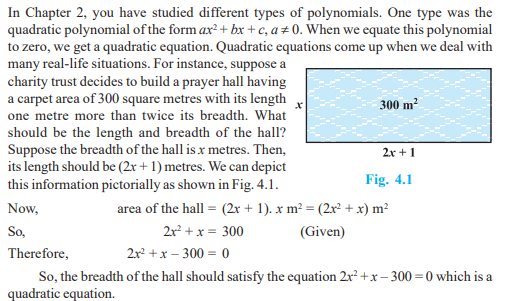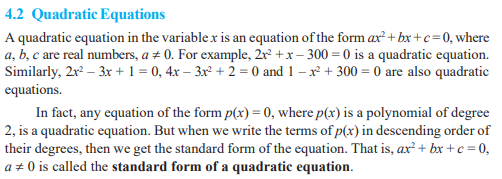• +91 9971497814
• info@interviewmaterial.com

### Related SubjectsMany people believe that Babylonians were the first to solve quadratic equations.For instance, they knew how to find two positive numbers with a given positive sumand a given positive product, and this problem is equivalent to solving a quadraticequation of the form x2 – px + q = 0. Greek mathematician Euclid developed ageometrical approach for finding out lengths which, in our present day terminology,are solutions of quadratic equations. Solving of quadratic equations, in general form, isoften credited to ancient Indian mathematicians. In fact, Brahmagupta (A.D.598–665)gave an explicit formula to solve a quadratic equation of the form ax2 + bx = c. Later,
Sridharacharya (A.D. 1025) derived a formula, now known as the quadratic formula,(as quoted by Bhaskara II) for solving a quadratic equation by the method of completingthe square. An Arab mathematician Al-Khwarizmi (about A.D. 800) also studiedquadratic equations of different types. Abraham bar Hiyya Ha-Nasi, in his book‘Liber embadorum’ published in Europe in A.D. 1145 gave complete solutions ofdifferent quadratic equations.In this chapter, you will study quadratic equations, and various ways of findingtheir roots. You will also see some applications of quadratic equations in daily lifesituations.Todays Deals### Chapter 4- Quadratic Equations Contributorskrishan

Name:
Email:

# Latest News# 9000 interview questions in different categories